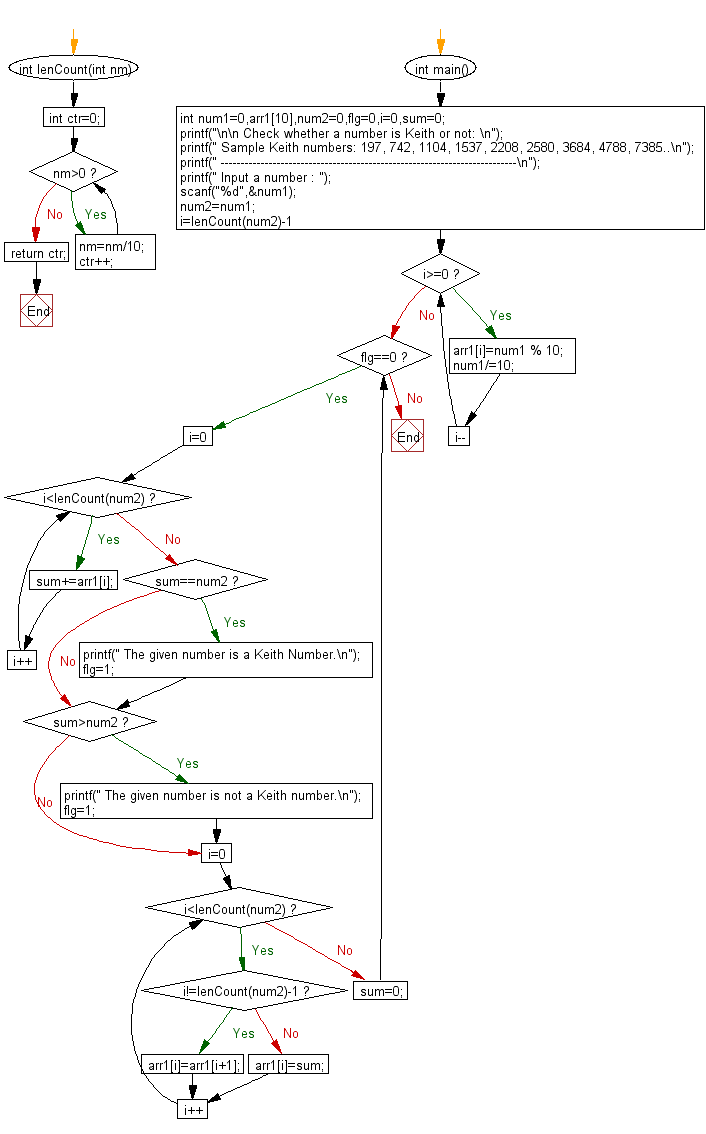﻿ C Program: Check if a number is Keith or not - w3resource# C Exercises: Check if a number is Keith or not

## C Numbers: Exercise-36 with Solution

Write a program in C to check if a number is Keith or not.

Test Data
Input a number : 1104

Sample Solution:

C Code:

``````# include <stdio.h>
# include <stdlib.h>

int lenCount(int nm)
{  int ctr=0;
while(nm>0)
{
nm=nm/10;
ctr++;
}
return ctr;
}

int main()
{
int num1=0,arr1,num2=0,flg=0,i=0,sum=0;
printf("\n\n Check whether a number is Keith or not: \n");
printf(" Sample Keith numbers: 197, 742, 1104, 1537, 2208, 2580, 3684, 4788, 7385..\n");
printf(" --------------------------------------------------------------------------\n");
printf(" Input a number : ");
scanf("%d",&num1);
num2=num1;
for(i=lenCount(num2)-1;i>=0;i--)
{
arr1[i]=num1 % 10;
num1/=10;
}
while(flg==0)
{
for(i=0;i<lenCount(num2);i++)
sum+=arr1[i];
if(sum==num2)
{
printf(" The given number is a Keith Number.\n");
flg=1;
}
if(sum>num2)
{
printf(" The given number is not a Keith number.\n");
flg=1;
}
for(i=0;i<lenCount(num2);i++)
{
if(i!=lenCount(num2)-1)
arr1[i]=arr1[i+1];
else
arr1[i]=sum;
}
sum=0;
}
}
```
```

Sample Output:

``` Input a number : 1104
The given number is a Keith Number.
```

Pictorial Presentation:Flowchart:C Programming Code Editor:

What is the difficulty level of this exercise?

Test your Programming skills with w3resource's quiz.

﻿

## C Programming: Tips of the Day

Why do C and C++ compilers allow array lengths in function signatures when they're never enforced?

It is a quirk of the syntax for passing arrays to functions.

Actually it is not possible to pass an array in C. If you write syntax that looks like it should pass the array, what actually happens is that a pointer to the first element of the array is passed instead.

Since the pointer does not include any length information, the contents of your [] in the function formal parameter list are actually ignored.

Ref : https://bit.ly/3fhlvdH